• 1D CNN，kernel 在 1 个方向移动，输入和输出是 2 维的，通常用于时序（Time-Series）数据
• 2D CNN，kernel 在 2 个方向移动，输入和输出是 3 维的，通常用于图像数据
• 3D CNN，kernel 在 3 个方向移动，输入和输出是4 维的，通用用于 3D 图像数据

## 1D 卷积 - Conv1D

Conv1D，kernel 沿着一个维度进行滑动.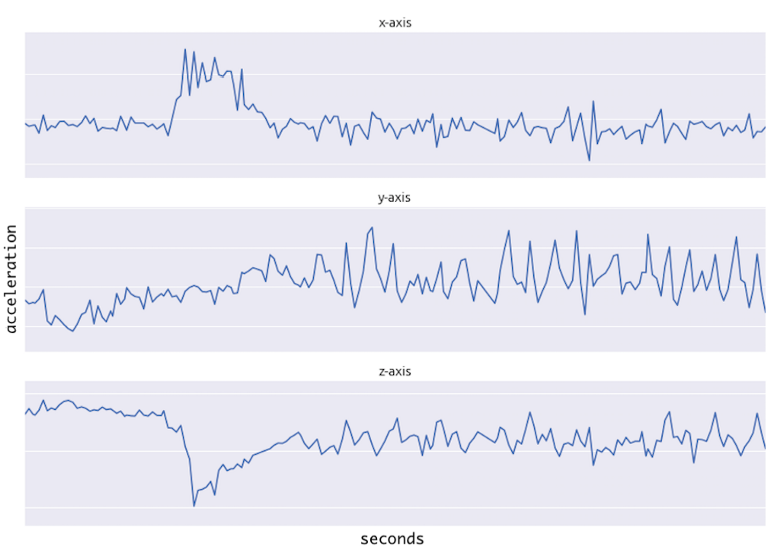kernel 在传感器数据上滑动的方式如下图，每一行表示某一轴的时间序列传感器数据. kernel仅能在时间轴方向上移动.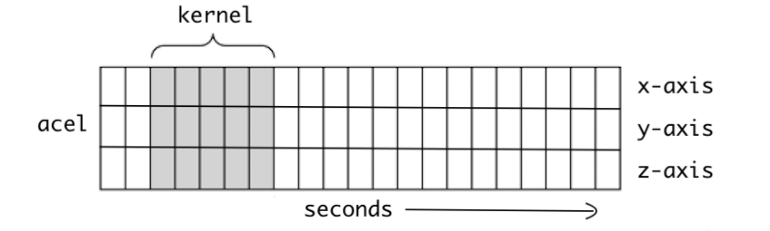'''
https://pytorch.org/docs/stable/generated/torch.nn.Conv1d.html
'''
import torch
from torch import nn

model = nn.Conv1d(in_channels=1, out_channels=10, kernel_size=5, stride=1)

input = torch.randn(20, 1, 50)
output = model(input)

1D CNNs 被普遍应用于传感器数据，还可以用于音频和文本数据，因为其可以被表示为时序数据. 如图：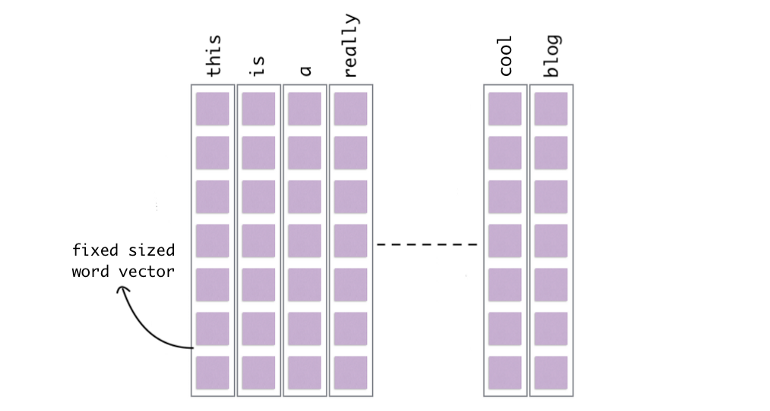## 2D 卷积 - Conv2D

Lenet-5 结构中首先提出标准CNN网络. Conv2D 普遍应用于图像数据. 其 2D 是因为，kernel 沿着数据的两个维度进行滑动，如图：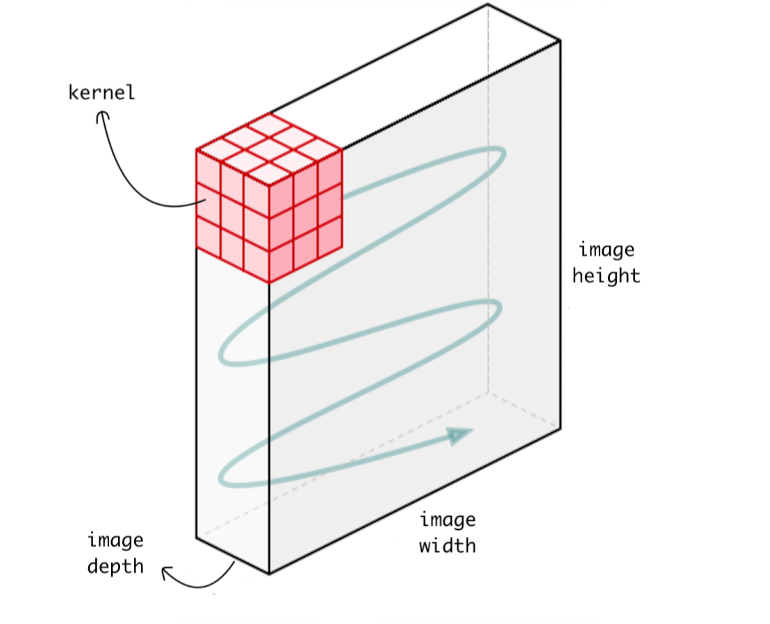CNN 的优势在于，采用 kernel 可以提取数据的空间特征. 例如，CNN 可以检测边缘、颜色分布等，其有助于网络对于图像分类的鲁棒性.

'''
https://pytorch.org/docs/stable/generated/torch.nn.Conv2d.html
'''
import torch
from torch import nn

# With square kernels and equal stride
model = nn.Conv2d(16, 33, 3, stride=2)

# non-square kernels and unequal stride and with padding
model = nn.Conv2d(16, 33, (3, 5), stride=(2, 1), padding=(4, 2))

# non-square kernels and unequal stride and with padding and dilation
model = nn.Conv2d(16, 33, (3, 5), stride=(2, 1), padding=(4, 2), dilation=(3, 1))

input = torch.randn(20, 16, 50, 100)
output = model(input)

## 3D 卷积 - Conv3D

3D CNN 中，kernel 沿 3 个维度滑动，如图：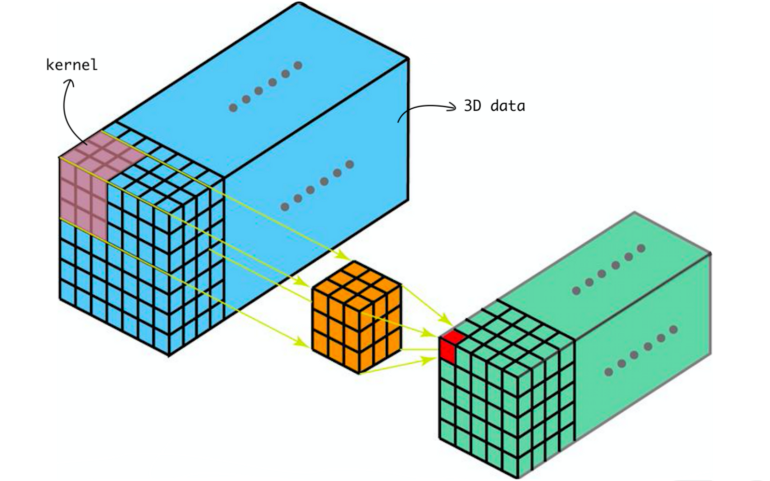Conv3D 通常应用于 3D图像数据，如，MRI （Magnetic Resonance Imageing） 数据、CT（Computerized Tomography）扫描数据等.

'''
https://pytorch.org/docs/stable/generated/torch.nn.Conv3d.html
'''
import torch
from torch import nn

# With square kernels and equal stride
model = nn.Conv3d(16, 33, 3, stride=2)

# non-square kernels and unequal stride and with padding
model = nn.Conv3d(16, 33, (3, 5, 2), stride=(2, 1, 1), padding=(4, 2, 0))

input = torch.randn(20, 16, 10, 50, 100)
output = model(input)
Last modification：February 11th, 2022 at 06:34 pm